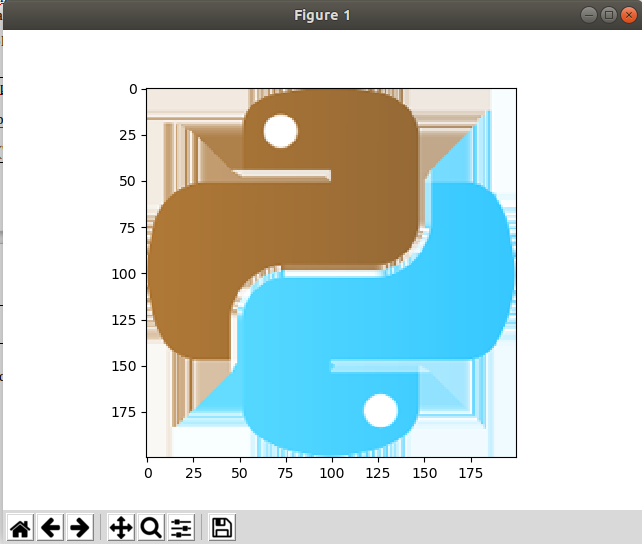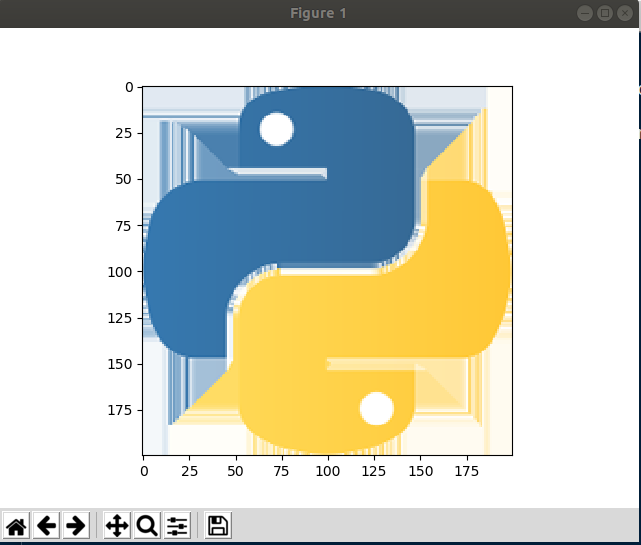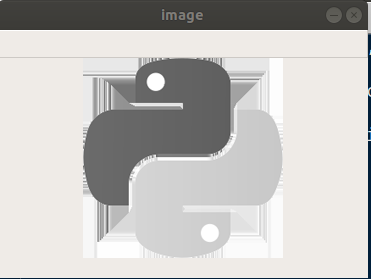## Transformation with OpenCV in Python

##### In this part of Learning Python we Cover Transformation with OpenCV In Python
Written by |29-Apr-2019 | 0 Comments | 290 Views

In this section, we will discuss how to get the feed of an IP camera. Most people do not have a webcam on their PC, but all have a smartphone, so it’s time to use smartphone smartly. Have you ever thought to get the camera feed in your computer, you can do lots of stuff with that like screencast it etc.

However, before jumping into the code, we have to install something on our mobile phone as well. Go to the google play store or app store and download IP Webcam the logo of that application looks like this:Figure 1

After downloading open the application and scroll down to the bottom where there is an option of starting a server. At the bottom of the screen after the camera opens there will be a URL which may look like this http://10.*.*.125:8080:

`import urllib.request`
`import cv2`
`import numpy as np`

`img_url='http://10.*.*.125:8080/shot.jpg'`

`while True:`
`    with urllib.request.urlopen(img_url) as url:`
`        response = url.read()`
`    array=np.array(bytearray(response), dtype=np.uint8)`
`    img=cv2.imdecode(array, -1)`

`    cv2.imshow('frame',img)`
`    if ord('q')==cv2.waitKey(1):`
`        break`

`cv2.destroyAllWindows()`

After importing all the modules, we made a variable or img_url in which the URL that we have copies was paste and added /shot.jpg. This the full URL of the frame that we will get. These frames will be continuous means frame will come one after the other, so we started an infinite while loop which loops over the frame till the camera is in running position. Then just like in the case of an image from the link we open the URL and extract the bytes and then decode the bytes to an image array so the python open cv can recognize it.

Finally, when a user wants to stop, he will press q and loop will be break window will be destroyed.

This feature enables anyone to analyze live webcam feed if he does not a webcam. Moreover, if you have a spare mobile, you can make your own security system by mounting the mobile somewhere no one can see it. However, it should not be used unethically.

Moreover, this will work only on the same LAN not on internet layer so your mobile and PC must be connected to the same WIFI.

Transformation:

BGR to RGB:

As we have discussed earlier, the OpenCV convert the image when loaded into BGR, but mostly the like matplotlib cater it as RGB which make the color difference, so we have to convert image from BGR to RGB.

Let’s take an example to plot the image loaded from OpenCV and then plotted on matplotlib:

`import numpy as np`
`import cv2`
`import matplotlib.pyplot as plt`

`img = cv2.imread('logo.png')`
`plt.imshow(img)`
`plt.show()`

The generated result can be seen in figure 2:Figure 2

As we can see colors are distorted. So, to convert BGR to RGB following method is applied:

`import numpy as np`
`import cv2`
`import matplotlib.pyplot as plt`

`img  = cv2.imread('logo.png')`
`img = cv2.cvtColor(img,cv2.COLOR_BGR2RGB)`
`plt.imshow(img)`
`plt.show()`

After loading the image, we call OpenCV CVT Color function which converts the image to RGB now if we plot the image look like this again:Figure 3

Transforming to grayscale:

Grayscale is another transformation in which we convert the color image to grayscale. First, we will see how to do this and then we will examine what happened when we convert the image to grays scale.

`import numpy as np`
`import cv2`
`import matplotlib.pyplot as plt`

`img  = cv2.imread('logo.png')`
`img = cv2.cvtColor(img,cv2.COLOR_BGR2GRAY)`
`cv2.imshow('image',img)`
`cv2.waitKey(0)`

So, the image that is generated is as follows:Figure 4

So now we will see what happened by printing the image before the conversion and after the conversion:

`import numpy as np`
`import cv2`
`import matplotlib.pyplot as plt`

`img  = cv2.imread('logo.png')`
`print("Before")`
`print()`
`print(img)`
`img = cv2.cvtColor(img,cv2.COLOR_BGR2GRAY)`
`print("After")`
`print()`
`print(img)`

Also, the output looks like this:

 Before   [[[242 233 225]   [242 233 225]   [242 233 225]   ...   [248 253 255]   [248 253 255]   [248 253 255]]    [[242 233 225]   [242 233 225]   [242 233 225]   ...   [248 253 255]   [248 253 255]   [248 253 255]]    [[242 233 225]   [242 233 225]   [242 233 225]   ...   [248 253 255]   [248 253 255]   [248 253 255]]    ...    [[250 248 244]   [250 248 244]   [250 248 244]   ...   [240 251 255]   [240 251 255]   [240 251 255]]    [[250 248 244]   [250 248 244]   [250 248 244]   ...   [240 251 255]   [240 251 255]   [240 251 255]]    [[250 248 244]   [250 248 244]   [250 248 244]   ...   [240 251 255]   [240 251 255]   [240 251 255]]]   After   [[232 232 232 ... 253 253 253]  [232 232 232 ... 253 253 253]  [232 232 232 ... 253 253 253]  ...  [247 247 247 ... 251 251 251]  [247 247 247 ... 251 251 251]  [247 247 247 ... 251 251 251]]

Before converting each pixel is represented as a list of 3 elements. Now the question arises why there are three elements. The three elements represent Red Blue and Green respectively after the conversion the 3-channel image is converted into one channel image now each nested list represent the rows of pixel and rows contain only the value that is either closer to white or closer to black. It is what happens when we convert the image to grayscale its pixels get converted from 3 channels to 1 channel.

Resizing The image:

Open cv provides the functionality to resize the image. The resize image function takes two parameters. 1st the object of the image and second the tuple of width and height. Following is the code to resize the image.

`import numpy as np`
`import cv2`
`import matplotlib.pyplot as plt`

`img  = cv2.imread('logo.png')`
`img = cv2.cvtColor(img,cv2.COLOR_BGR2GRAY)`
`img = cv2.resize(img,(10,10))`
`print(img)`

We had seen earlier that when we printed the image, a huge array was formed that was not even able to printed fully, but when we resize the image the array will be printed fully on screen as follows:

 [[232 232 232 102 100  98  96 231 231 253]  [195 182 117 255 100  98  96 162 215 253]  [195 186 147 144 143  98  96 163 218 253]  [106 105 103 101 100  98  96 166 202 201]  [106 105 103 101 100  98  96 203 202 201]  [106 105 117 211 209 207 206 204 202 201]  [106 105 219 211 209 207 206 204 202 201]  [247 186 216 211 209 234 232 232 231 251]  [247 195 217 211 209 207 255 225 229 251]  [247 254 254 211 209 207 206 251 251 251]]

There are 10 rows and 10 columns; each element represents one pixel.